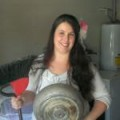# E is for Equals

When I was brainstorming an idea for our next preschool unit, I mentioned doing E is for equals to my husband – the math teacher. His first response, “Only if you teach her that equals means the same value is on both sides.” A lot of elementary students think that the equal sign means “the answer goes here”, instead of “this side is the same as that side”. With that little bit of professional advice, I started in on the E is for Equals unit with my preschooler.

Objective 1: To help the children understand that the equal sign (=) represents the word equals.
Objective 2: To help the children understand that equals means the same on both sides.

Materials: One E is for Equals printable for each child.

One bag of popcorn—popped
Two plates

Vocabulary: Equals – meaning to be the same on both sides of the equation.
Equation – meaning a number sentence.

Visual Vocabulary: = (the equal sign) – meaning equals.

Method:
Begin by explaining to the child that the equal sign means the word equals. Then explain that equals means the two sides are the same. Finally, explain that in math, we place numbers in sentences that tell us something. Those sentences are called equations. And the equal sign is used to show that what’s on one side of the equation (number sentence) is the same as what’s on the other side.

Use the worksheet to show the children the equal sign and to talk about how one side of the equal side need to be the same as the other side of the equal sign.

Now, it’s time to take this theoretical discussion and apply it in a way the children can understand it. This is where the popcorn and plates come in.

Our first batch of popcorn for discussion.

Place a plate on either side of the equal sign. Ask the children some of the following questions:

Are the plates equal?
Are both sides of the equal sign the same?
How do you know both sides are the same?

Then place some popcorn on one plate, but not the other. And ask the questions again.

Ask the children to make the equation true, (make the equation the same) by adding or subtracting popcorn from one of the plates.

Counting the popcorn, and making the two side the same (or equals).

Keep repeating the process until the children seem very confident in their understanding of when the equation is equal (or true). Be sure to reinforce the vocabulary in this unit by using in throughout the discussion and activity. This will help the children gain fluent use of the vocabulary words.

To make the activity more fun, and more interactive, at this point start having the children quiz the care giver and set up the popcorn equations. Teaching a concept is one of the best ways to reinforce the concept, and teaching a grownup is always super fun.

An equation my preschooler set up for me to solve.

After everyone has tired of the activity, compliment the children on their hard work and tell them how much fun you had. Then, enjoy what popcorn hasn’t been handled.

Here is a link to a reinforcement activity to help children further solidify their understanding that E is for Equals.

1.1.Post
Author
2.1.Post
Author
3.This is a great idea. Your husband’s point is something I have not thought about. I can see how a child would think it meant that the ‘answer goes here.’

1.Post
Author
4.This is so great! And I love the idea of teaching what equals really means… I think with the way our school systems are going that theory may be on it’s way out but it is so true. Once again printing and going to start with my boys when they get a little older 🙂

1.Post
Author
5.1.Post
Author